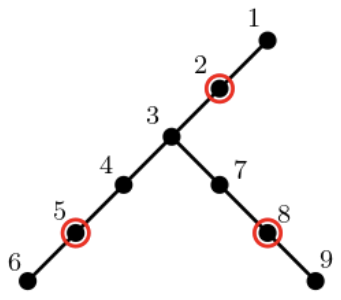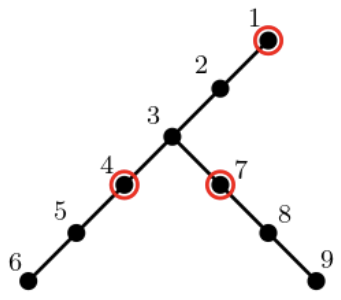시간 제한메모리 제한제출정답맞힌 사람정답 비율
1 초 (추가 시간 없음) 1024 MB197633937.864%

## 문제

KOPANG is one of largest online vendors in Korea and introduced so called “early-morning delivery” for the first time. To cope with the growing demand, KOPANG plans to build new logistical warehouses. The locations of logistical warehouses must be within a certain distance from customers to keep the delivery time guaranteed by KOPANG to the customers.

The logistics network is modelled as a connected tree $T$. Each node of $T$ represents a region such as a city or a province in Korea, and each edge of $T$ represents a transportation road connecting two regions. KOPANG wants to select one or more nodes of $T$ satisfying the distance restriction for the logistical warehouses. Before the selection, KOPANG first fixed a distance parameter $K$ through sufficient research. KOPANG now wants to select the minimum number of nodes satisfying the distance restriction that the distance from every node of $T$ to its closest selected node (warehouse) is at most $K$. The distance of two nodes $u$ and $v$ is the number of edges of the (unique) path in $T$ that connects $u$ and $v$. Note that the distance is defined as zero if $u = v$.

For example, Figure G.1 below shows a tree $T$ with nine nodes and eight edges. For $K = 1$, if three warehouses are located at nodes $2$, $5$, and $8$, marked with red circles as in Figure G.1 (a), then the distance of every node of $T$ to the closest warehouse is at most one. Two warehouses are not enough to satisfy the distance restriction, so three warehouses are the minimum. For $K = 2$, three warehouses are still required; warehouses at nodes $2$, $5$, and $8$ for $K = 1$ are the ones for $K = 2$. Of course, the locations of the minimum number of warehouses are not unique; three warehouses at nodes $4$, $7$, and $1$ as in Figure G.1 (b) also satisfy the distance restriction for $K = 2$.

Given a connected tree $T$ and a positive integer $K$, write a program to select the minimum number of nodes (warehouses) of $T$ satisfying the distance restriction, that is, the distance of every node of $T$ to its closest warehouse is at most $K$.(a) (b)

Figure G.1 The nodes marked with red circles are the ones selected for warehouses.

## 입력

Your program is to read from standard input. The input starts with a line containing two integers $n$ and $K$ ($1 ≤ K ≤ n ≤ 10^5$), where $n$ is the number of nodes in a connected tree and the maximum distance from each node in the tree to its closest selected node is at most $K$. In the following $n-1$ lines, the edge information is given; the $i$-th line contains two positive integers representing two indices of the end nodes of the $i$-th edge. The nodes are indexed from $1$ to $n$.

## 출력

Your program is to write to standard output. Print exactly one line that contains the minimum number of the selected nodes for logistical warehouses satisfying the distance restriction for the given tree and the distance parameter $K$.

## 예제 입력 1

9 1
2 1
7 3
3 4
4 5
6 5
7 8
3 2
8 9


## 예제 출력 1

3


## 예제 입력 2

9 2
2 1
7 3
3 4
4 5
6 5
7 8
3 2
8 9


## 예제 출력 2

3


## 예제 입력 3

9 8
2 1
7 3
3 4
4 5
6 5
7 8
3 2
8 9


## 예제 출력 3

1


## 힌트

The first two samples correspond to Figure G.1 (a) and (b), respectively.